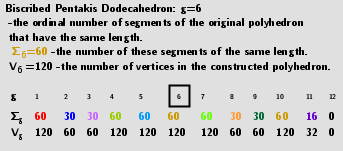# Polyhedron(V=120) from Biscribed Pentakis Dodecahedron for the case of trisection of its 6th-order segments

A polyhedron is constructed whose V=120 vertices are the points of the trisection of the segments the same length 6th-order(g=6) of the Biscribed Pentakis Dodecahedron. Geometric Constructions are in Applet: Series of polyhedra obtained by trisection (truncation) different segments of the original polyhedron, and the resulting polyhedra in Applet: Serie of polyhedra obtained by trisection (truncation) segments of the Biscribed Pentakis Dodecahedron.